# Voltage source:

Let see, the definition of Voltage source, current source, ideal voltage sources, ideal current sources, independent voltage sources, and independent current sources.

## Voltage source: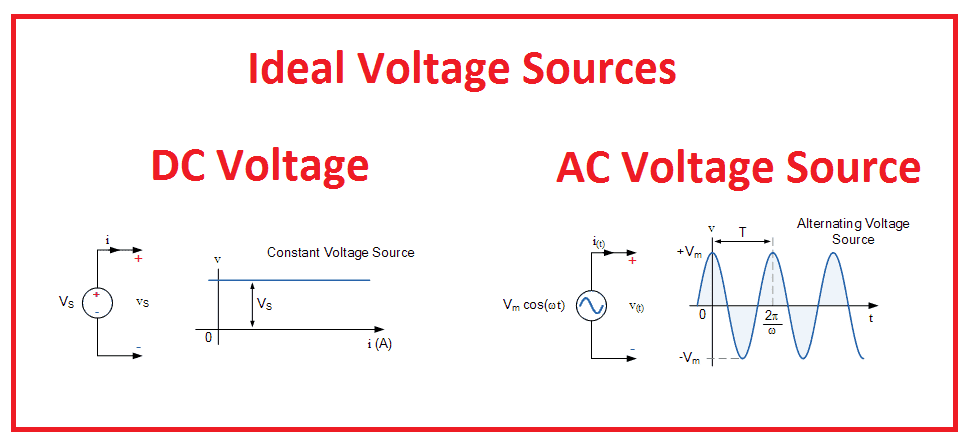## Ideal Voltage source:

A voltage source whose output voltage remains constant, at any load current or fixed voltage at changes in load is known as an ideal voltage source. The internal resistance of an ideal voltage source is zero.  But in practice, we can not construct ideal voltage source, because of the without internal resistance we cannot build a voltage source. Also in real-world voltage source cannot supply unlimited current. A voltage source is the dual of a current source. Real-world sources of electrical energy, such as batteries, generators, and power systems, can be modeled for analysis purposes as a combination of an ideal voltage source and additional combinations of impedance elements.

Two types of voltage source:

1. Dependent Voltage source
2. Independent voltage source

## Dependent Voltage source:

Independent voltage sources, the source voltage depends on other voltage or current sources in the same circuit or network. The dependent voltage source has four different types.

1. Voltage controlled voltage source.
2. Current controlled Voltage source.

### Voltage controlled voltage source:

The source of the circuit delivers the output voltage as per the voltage of the dependent element

Example: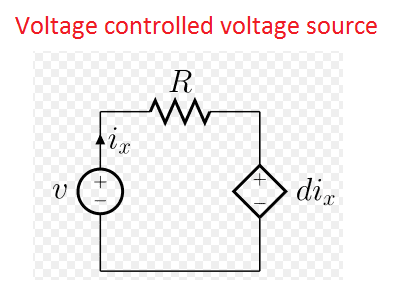### Voltage controlled Current source.

The source delivers the current (flow of electron) as per the voltage of the dependent element in the same network

Example:### Current Controlled current source.

The source delivers current as per the current of the dependent element in the circuit.

Example:

### Current controlled voltage source:

The source delivers Voltage as per the current of the dependent element in the circuit.

Example: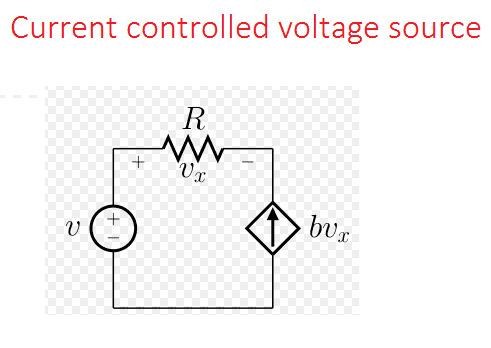Note: The sign or polarity of each dependent element to be considered.

## Independent voltage source:

Independent voltage is nothing but the source voltage does not depend on either the magnitude or direction of the current flowing through the source.

Example: Battery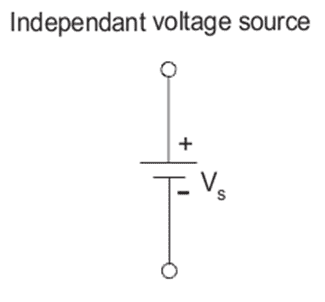## Series connected independent voltage sources:

Let see the output voltage of series connected three battery V1, V2, and V3: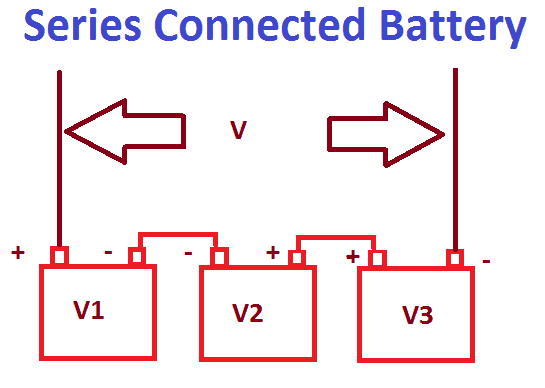Apply Kirchhoff’s Voltage Law: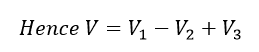Hence the total output voltage is equal to the algebraic sum of all individual voltage sources.

Example: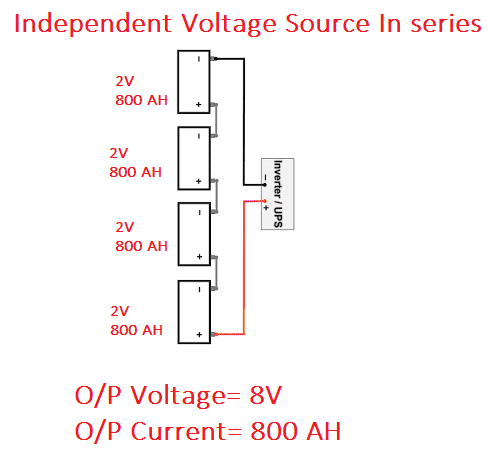### Application:

1. This technic is mainly used in battery storage system, i.e UPS system. To build a 320 V DC Voltage, we have to connect individual single cell with the output voltage of 2 V each and 160 numbers batteries in series.
2. In the same way, to get 110 VDC we need to connect 55 batteries in series. 110 VDC is used in all electrical switchgear controls. Example: Star delta wiring, Circuit breaker internal and external wiring, Turbine control wiring, emergency pumps etc.

### Key points:

• In series connected independent voltage sources, the output voltage will increase and the total current is equal to one of the battery capacity (rated current). i.e consider above case the 2-volt battery has 800 AH (Ampere hour), if 160 number of battery is connected in series means the total output voltage is 320 VDC, and the output current is 800 AH.
• All the battery should be identical. Do not connect the battery with low rating (low AH) and higher AH battery. Then output AH will get decreased and the total rating may decrease to low rating. i.e in above case, out of 160 numbers, one battery is replaced by 2V, 400 AH, then the total rating will get reduced to 320 V, 400 AH
Learn More:   LED Light Power Consumption Calculation & LED Energy Bill Calculator

## Parallel connected independent voltage sources:

When you connect all the battery in parallel means, the output current will get increase but the output voltage remains same (rated voltage of the single battery).

Example: 3 numbers of 12 Volts, 100 AH battery is connected in parallel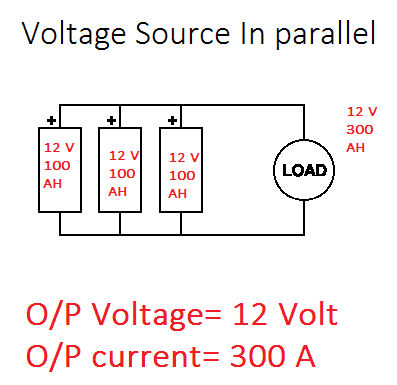Output voltage => 12 Volts

Output Current => 300 AH

Note: All the battery’s output voltage should be equal and the current is not required to be in rating.

Application: This method is used to start heavy engine like diesel generator’s starter.